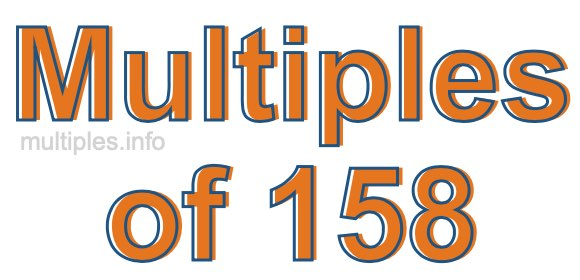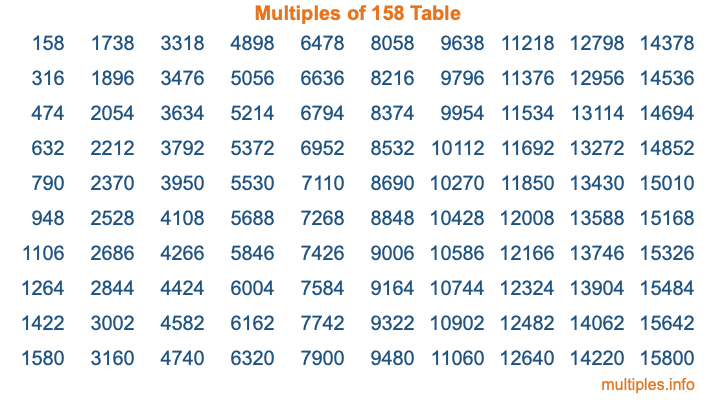Multiples of 158Welcome to the Multiples of 158 page. Here we will first teach you everything you will ever need to know about the multiples of 158, and then give you a study guide summary of everything we taught you to make sure you remember it all. Use this page to look up facts and learn information about the multiples of 158. This page will make you a multiples of one hundred fifty-eight expert!

Definition of Multiples of 158
Multiples of 158 are all the numbers that when divided by 158 equal an integer. Each of the multiples of 158 are called a multiple. A multiple of 158 is created by multiplying 158 by an integer.

Therefore, to create a list of multiples of 158, you start with 1 multiplied by 158, then 2 multiplied by 158, then 3 multiplied by 158, and so on for as long as you want. Thus, the list of the first five multiples of 158 is 158, 316, 474, 632, and 790. To see a larger list of multiples of 158, see the printable image of Multiples of 158 further down on this page. We also have a category where you can choose any nth multiple of 158.

Multiples of 158 Checker
The Multiples of 158 Checker below checks to see if any number of your choice is a multiple of 158. In other words, it checks to see if there is any number (integer) that when multiplied by 158 will equal your number. To do that, we divide your number by 158. If the the quotient is an integer, then your number is a multiple of 158.

Is  a multiple of 158?

Least Common Multiple of 158 and ...
A Least Common Multiple (LCM) is the lowest multiple that two or more numbers have in common. This is also called the smallest common multiple or lowest common multiple and is useful to know when you are adding our subtracting fractions. Enter one or more numbers below (158 is already entered) to find the LCM.

Check out our LCM Calculator if you need more details about the Least Common Multiple or if you need the LCM for different numbers for adding and subtraction fractions.

nth Multiple of 158
As we stated above, 158 is the first multiple of 158, 316 is the second multiple of 158, 474 is the third multiple of 158, and so on. Enter a number below to find the nth multiple of 158.

th multiple of 158

Multiples of 158 vs Factors of 158
158 is a multiple of 158 and a factor of 158, but that is where the similarities end. All postive multiples of 158 are 158 or greater than 158. All positive factors of 158 are 158 or less than 158.

Below is the beginning list of multiples of 158 and the factors of 158 so you can compare:

Multiples of 158: 158, 316, 474, 632, 790, etc.

Factors of 158: 1, 2, 79, 158

As you can see, the multiples of 158 are all the numbers that you can divide by 158 to get a whole number. The factors of 158, on the other hand, are all the whole numbers that you can multiply by another whole number to get 158.

It's also interesting to note that if a number (x) is a factor of 158, then 158 will also be a multiple of that number (x).

Multiples of 158 vs Divisors of 158
The divisors of 158 are all the integers that 158 can be divided by evenly. Below is a list of the divisors of 158.

Divisors of 158: 1, 2, 79, 158

The interesting thing to note here is that if you take any multiple of 158 and divide it by a divisor of 158, you will see that the quotient is an integer.

Multiples of 158 Table
Below is an image of the first 100 multiples of 158 in a table. The table is in chronological order, column by column. The first column has the first ten multiples of 158, the second column has the next ten multiples of 158, and so on.The Multiples of 158 Table is also referred to as the 158 Times Table or Times Table of 158. You are welcome to print out our table for your studies.

Negative Multiples of 158
Although not often discussed or needed in math, it is worth mentioning that you can make a list of negative multiples of 158 by multiplying 158 by -1, then by -2, then by -3, and so on, to get the following list of negative multiples of 158:

-158, -316, -474, -632, -790, etc.

Multiples of 158 Summary
Below is a summary of important Multiples of 158 facts that we have discussed on this page. To retain the knowledge on this page, we recommend that you read through the summary and explain to yourself or a study partner why they hold true.

There are an infinite number of multiples of 158.

A multiple of 158 divided by 158 will equal a whole number.

158 divided by a factor of 158 equals a divisor of 158.

The nth multiple of 158 is n times 158.

The largest factor of 158 is equal to the first positive multiple of 158.

158 is a multiple of every factor of 158.

158 is a multiple of 158.

A multiple of 158 divided by a divisor of 158 equals an integer.

158 divided by a divisor of 158 equals a factor of 158.

Any integer times 158 will equal a multiple of 158.

Multiples of a Number
Here you can get the multiples of another number, all with the same attention to detail as we did for multiples of 158 on this page.

Multiples of
Multiples of 159
Did you find our page about multiples of one hundred fifty-eight educational? Do you want more knowledge? Check out the multiples of the next number on our list!